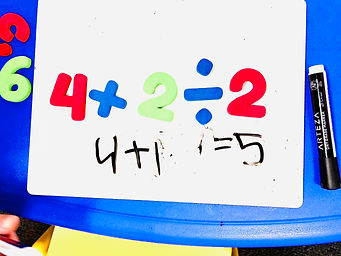## Ms. AJ

### Target 1​

###### Lesson Type:

Continuation

Number Operation

:

Computation

Subtract within 1,000 (regrouping limited to only one place-value).

###### 1:

Be introduced to a variety of computation strategies for subtracting within 1,000 and select a strategy that works best.

###### 2:

Understand why the standard algorithm for subtraction is based on place-value.

###### 3:

Understand that when subtracting a multi-digit number, each place-value is being subtracted individually to create the new number (ones are subtracted from ones, tens are subtracted from tens, etc.)

###### 4:

Understand that when adding a multi-digit number, to solve start in the lowest place-value and go left.

###### 5:

Understand that the same process is utilized to subtract three or more whole numbers that is used to subtract only two numbers.

2nd

###### Vocabulary:

Subtraction, Borrowing, Regroup

Activities:

Students practiced solving two and three-digit subtraction problems which included regrouping, drawing a "regrouping house" to help them visualize the problems.

Students played "Subtraction War", in which they took turns picking two cards at random from the top of their decks, subtracting their two cards (bigger number by smaller number; finding the difference), and determining who had the lowest number. The person with the lowest number wins all the cards in the round. If they get the same answer, they have a "War", in which three cards are placed facing down and they draw two more and flip those. Again, the one with the lowest number wins, but this time they win a total of 14 cards instead of only 4.### Home Exploration

###### Guiding Questions:## Absent Students:

Neha

### Target 2

:

###### 1:

Solve problems that have more than one operation.

###### 2:

Understand that the presence of parenthesis in an arithmetic equation signals for this part of the problem to be solved first.

###### 3:

Understand when solving a problem that has both addition and subtraction operations that solving starts on the left and continues to the right.

3rd

###### Vocabulary:

PEMDAS (Parenthesis, Exponents, Multiplication, Division, Addition, Subtraction), Order of Operations

Activities:

Students continued with "PEMDAS" and Order of Operations by doing a few problems in which various arithmetic was involved, as well as parenthesis.

Students started to explore "squares" (powers of two) through exponents.

Students created their own "Order of Operations" problems using number magnets, solved them, then switched with their classmates to solve each others'.### Home Exploration

###### Guiding Questions:### Target 3

:

###### Vocabulary:

Activities:### Home Exploration